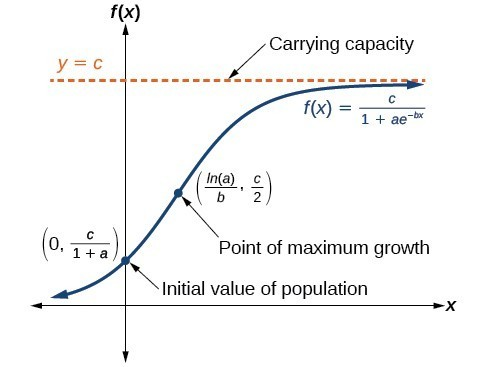## Use logistic-growth models

Exponential growth cannot continue forever. Exponential models, while they may be useful in the short term, tend to fall apart the longer they continue. Consider an aspiring writer who writes a single line on day one and plans to double the number of lines she writes each day for a month. By the end of the month, she must write over 17 billion lines, or one-half-billion pages. It is impractical, if not impossible, for anyone to write that much in such a short period of time. Eventually, an exponential model must begin to approach some limiting value, and then the growth is forced to slow. For this reason, it is often better to use a model with an upper bound instead of an exponential growth model, though the exponential growth model is still useful over a short term, before approaching the limiting value.

The logistic growth model is approximately exponential at first, but it has a reduced rate of growth as the output approaches the model’s upper bound, called the carrying capacity. For constants a, b, and c, the logistic growth of a population over time x is represented by the model

$f\left(x\right)=\frac{c}{1+a{e}^{-bx}}$

Figure 6 shows how the growth rate changes over time. The graph increases from left to right, but the growth rate only increases until it reaches its point of maximum growth rate, at which point the rate of increase decreases.Figure 6

### A General Note: Logistic Growth

The logistic growth model is

$f\left(x\right)=\frac{c}{1+a{e}^{-bx}}$

where

• $\frac{c}{1+a}$ is the initial value
• c is the carrying capacity, or limiting value
• b is a constant determined by the rate of growth.

### Example 5: Using the Logistic-Growth Model

An influenza epidemic spreads through a population rapidly, at a rate that depends on two factors: The more people who have the flu, the more rapidly it spreads, and also the more uninfected people there are, the more rapidly it spreads. These two factors make the logistic model a good one to study the spread of communicable diseases. And, clearly, there is a maximum value for the number of people infected: the entire population.

For example, at time = 0 there is one person in a community of 1,000 people who has the flu. So, in that community, at most 1,000 people can have the flu. Researchers find that for this particular strain of the flu, the logistic growth constant is = 0.6030. Estimate the number of people in this community who will have had this flu after ten days. Predict how many people in this community will have had this flu after a long period of time has passed.

### Solution

We substitute the given data into the logistic growth model

$f\left(x\right)=\frac{c}{1+a{e}^{-bx}}$

Because at most 1,000 people, the entire population of the community, can get the flu, we know the limiting value is = 1000. To find a, we use the formula that the number of cases at time = 0 is $\frac{c}{1+a}=1$, from which it follows that = 999. This model predicts that, after ten days, the number of people who have had the flu is $f\left(x\right)=\frac{1000}{1+999{e}^{-0.6030x}}\approx 293.8$. Because the actual number must be a whole number (a person has either had the flu or not) we round to 294. In the long term, the number of people who will contract the flu is the limiting value, = 1000.

### Analysis of the Solution

Remember that, because we are dealing with a virus, we cannot predict with certainty the number of people infected. The model only approximates the number of people infected and will not give us exact or actual values.

Figure 7 gives a good picture of how this model fits the data.

### Try It 5

Using the model in Example 5, estimate the number of cases of flu on day 15.

Solution